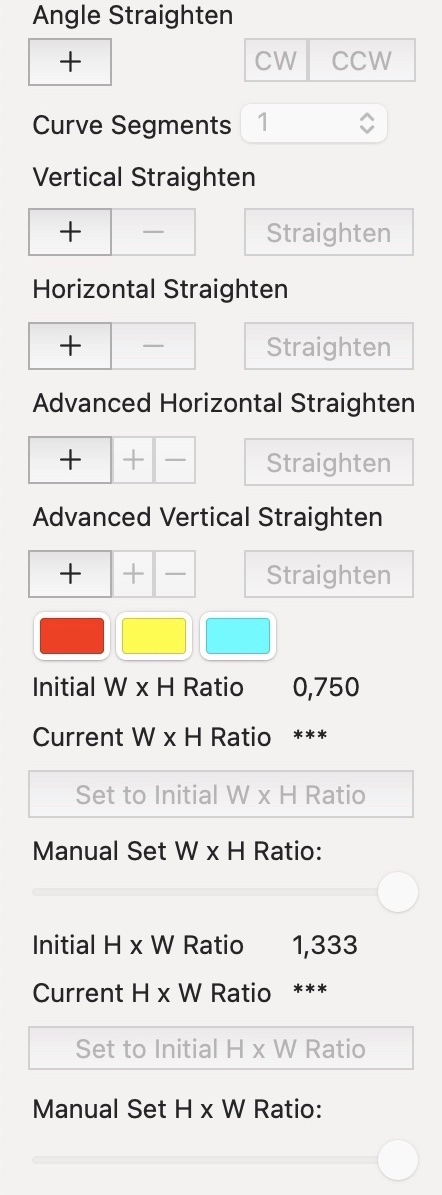# ToolbarThe "Angle Straighten" is for angle corrections on the image. Angle correction can be done in a clockwise or counterclockwise direction. The angle degree depends on the line slope on the image.

Curve Segments represent a number of independent curved segments in one curve. Segments are connected with anchor points. Every segment contains 4 points - 2 anchor points and 2 control points. Anchor points represent segment scope. Control points define segment curvature. The maximum number of curve segments is 50. A new possibility allows a change number of curve segments on a selected curve. If the number is increased, the curve maintains its shape with more possibility for precise adjustment. If the number is decreased, the curve will be recalculated to the nearest possible shape.

The "Vertical Straighten" is for vertical perspective corrections. Vertical straighten performs perspective corrections like an optic Tilt-Shift Lens. Vertical straighten is the perfect tool for barrel deformation correction on images taken with wide and ultra wide lenses.

The "Horizontal Straighten" is for horizontal corrections like pincushion deformation on images. Horizontal straighten is suitable for perspective correction of panoramic pictures. With a combination of Vertical and Horizontal straighten (or vice versa), successful correction can be accomplished on images taken with a Fish-eye lens.

Advanced Vertical and Advanced Horizontal Straighten are new tools developed to allow users to influence straighten perform. The user defines the range where straighten will occur by Border curves. There are 2 other curves called Guide and Leading curves. These 2 curves represent curve set in advanced straighten because Guide and Leading curves always do in pairs. The Guide curve represents the deformation line. The Leading curve is the user's way of how the Guide curve will be processed.

The "Ratio Adjustment' is for setting the final aspect ratio between the Width and Height of the corrected image. Button "Set to Initial W x H Ratio" rescales the image after Horizontal Straighten to the initial Width x Height ratio. Also button "Set to Initial H x W Ratio" rescales the image after Vertical Straighten to the initial Height x Width Ratio. User can manually set the Width x Height ratio after Horizontal Straighten and the Height x Width ratio after Vertical Straighten if ratio adjustment demands fine tuning.

Red, yellow and cyan squares are colour wells for curves colour. The user chooses a colour that is more contrasted against the image. Colour can be changed at any time.